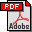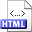An almost naive algorithm for finding relative neighbourhood graphs in Lp metrics Authors: Jyrki Katajainen and Olli Nevalainen Published in: Informatique Théorique et Applications 21,2 (1987), 199-215 Full text:PDF (726 kB) Copyright: © Gauthier-Villars Abstract: The relative neighbourhood graph (RNG) of a set of n points in a d-dimensional space contains an edge between a particular pair (v, w) of points if in the point set there is no other point for which the larger of distances from v and w is smaller than the distance of v and w.Let ℜpd denote the d-dimensional space with the Lp metric, 1 ≤ p ≤ ∞. A new simple algorithm for computing the RNG in ℜpd is given. It is an improved version of the O(d n2 + n3) algorithm proposed by R.B. Urquhart. In ℜp2 the worst case running time of our algorithm is O(n2.5) for 1 < p < ∞, for p = 1 or p = ∞ the worst case time is Θ(n3), and for point sets uniformly distributed in a unit square the average time is O(n2) for 1 ≤ p ≤ ∞. The demand for the storage space is O(d n + n2) and it can be reduced to O(d n + |RNG|), where |RNG| stands for the cardinality of the output, but this reduction manifolds the observed running time by a constant factor. Related:HTML (Thesis) BibLATEX: ```@article{KN1987J, author = {Jyrki Katajainen and Olli Nevalainen}, title = {An almost naive algorithm for finding relative neighbourhood graphs in \$L_p\$ metrics}, journaltitle = {Informatique Th\'{e}orique et Applications}, volume = {21}, number = {2}, year = {1987}, pages = {199--215}, } ```